Haili Chui's Research I recently completed my Ph.D. from Yale University. I now work at R2 Technologies, CA. My research interests include computer vision, image processing and computer graphics. I have also worked extensively on many medical image analysis problems. The following are a few topics that I have been working on. Recovering Non-Rigid Motion/Deformation Through Point Matching Many real-world problems require recovering non-rigid motions/deformations between objects represented by two images. If we can represent the objects as compact geometrical features extracted from the images, such as points, then we can find the desired deformation by solving the resulting point matching problem. Included below are a few examples for the point matching problem as well as its applications.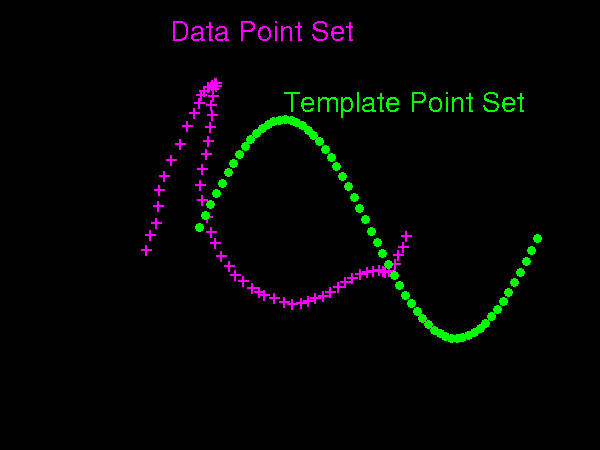Non-Rigid Point Matching: Example I Two point sets are shown here. We attempt to align these two point sets by deforming one set (template) onto the other set (data). Note that the correspondence between the two point sets is also unknown. We are solving for both the deformation and the correspondence together. See the matching process of our algorithm.Non-Rigid Point Matching: Example II Data points can be corrupted with noise and outliers. In order for the matching to be successful, the algorithm needs to "recognize" the outliers and reject them. See the matching process of our algorithm. Care to know more about non-rigid point matching? More details/demos here.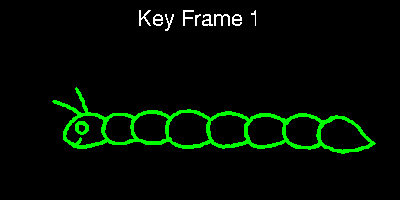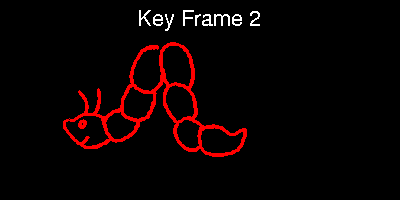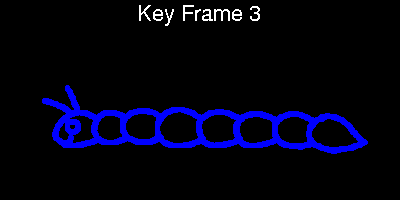Application I: Keyframe Animation: Three key frames are shown. We represent the object in each frame, which is a crawling caterpillar in this case, by points. Then we solve the point matching problem to find the non-rigid deformations between frames. See the estimated deformation between frame 1 and 2. A simple linear interpolation is then used to generate all the intermediate frames. These intermediate frames can form a full animated sequence. See the animated sequence.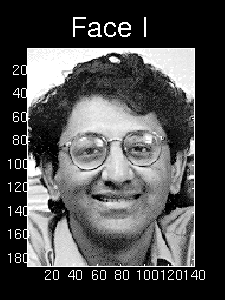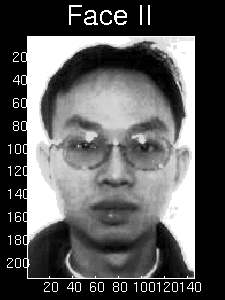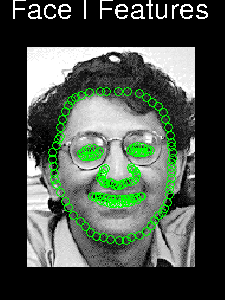Application II: Face Matching Two faces and their feature points are shown as an example. Through the matching of the two feature point sets, we can find the deformation that can warp the shape of one person's face to be the same as the other. Note that the points in the two sets do have to be exactly corresponding to each other. See the estimated deformation. See the warping of the face image. You may have noticed the interesting fact that the averaged face looks similar to both face I and II after the correct warping is applied. The animation of human figure motion or facial expressions certainly requires the modelling of complex non-rigid deformations. From the keyfram animation example and this face matching example, there is certainly potential of utilizing the non-rigid point matching algorithm further for such sophisticated animations.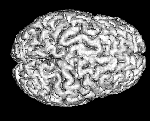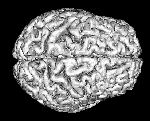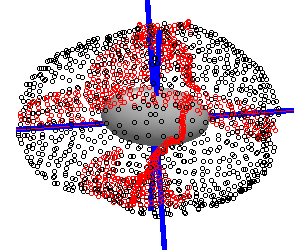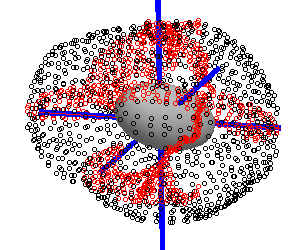Application III: Brain Anatomical Normalization Non-rigid alignment of brain images (e.g. MRI) are required for quantitative analysis. We have chosen a number of major brain structures as the feature surfaces (outer cortex) and feature ribbons (major sulci). Points are sampled from these surfaces/ribbons to form the feature point set. Then the appropriate deformation is found through point matching. See the 3D feature point set for brain 1. See the 3D feature point set for brain 2. For visulization, only two ribbons (left/right central sulcus) are shown. See the warping. This example also serves as an example of 3D non-rigid point matching.

 A More Efficient Way to Do Point Matching: Joint Clustering-Matching Matching thousands (or even more) points involves heavy computation in evaluating large correspondence matrices as well as high dimensional deformation functions. The algorithm can be made more efficient through sampling (e.g. clustering) and utilizing the fewer cluster centers as a rough representation for matching. However, the conventional way of perfoming matching separately after sampling often turns out to be not accurate enough. We devised a joint clustering (sampling) and matching algorithm that facilitate the feedback between these two processes, achieving optimal sampling result and improved matching while reducing the computational cost significantly.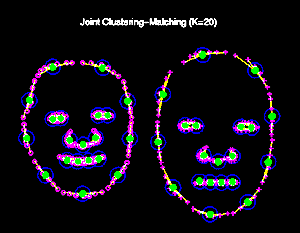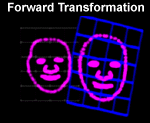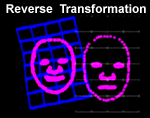Joint Clustering-Matching: Example Shown here is an example of matching two face feature point sets. The original point sets (around 150 in each set) are being clustered down to 20 cluster centers and matched at the same time. By jointly performing clustering and matching, the cluster centers are placed at the optimal positions that are consistent with each other. Note the cluster centers (green discs) are always located at corresponding positions in the point shape pattern (e.g. each face has 4 for the mouth, 3 for nose and etc.) The optimal placement of the cluster centers improves the accuracy of the alignment in spite of the fact that there are far fewer cluster centers (hence much faster) than the original points.

 Estimating An Average Shape: Matching Multiple Point Shape Sets Simultaneously The idea is inspired by the "shape average" problem, which can be briefly summarized as the following: given N (N>=2) sample shape patterns (e.g. point sets), compute the average shape for which joint distance between all the samples and the average is minimal. Based on the joint clustering-matching algorithm, we come up with a un-biased (all sample shapes are treated equally) average shape estimation algorithm that can align multiple sample point sets simultaneously, and compute an average shape point set as well.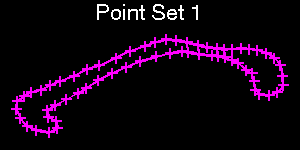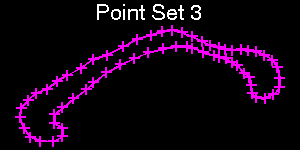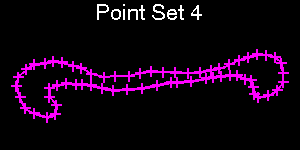......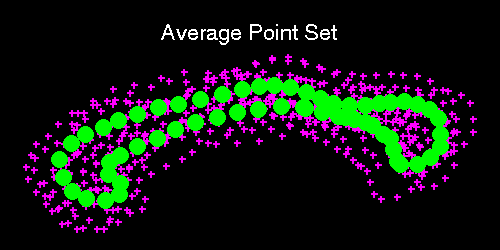Estimating An Average Point Shape: Example Shown here is an example of estimating an average shape from nine (only 4 are shown on the left) sample shape point-sets. The correspondences of the sample points are unknown. The algorithm finds the correspondences of the sample points, and computes the average shape point set. See all the sample shapes and the average shape. See all the deformations found between the samples and the average.

Comments?   Email me.